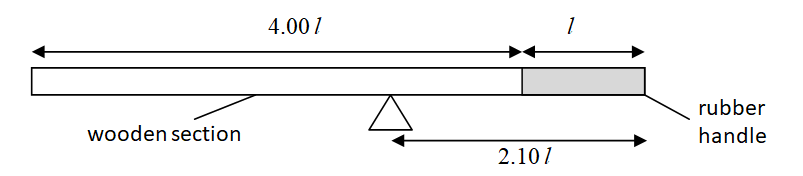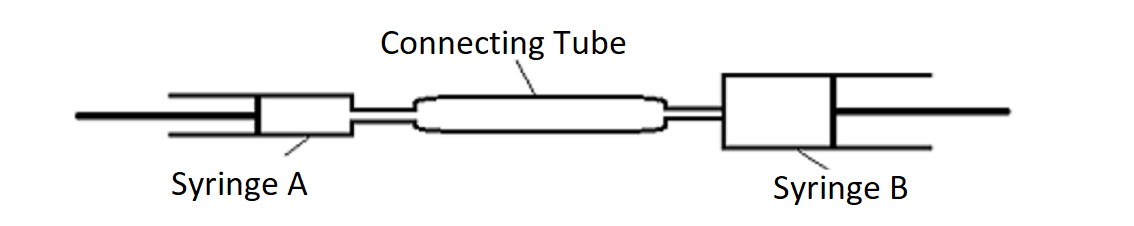# Check Your Understanding of Physics Concepts

FEATURED

## Physics Question Guide

Go through some of these questions and check your understanding of physics concepts

#### Topics: Turning Effect of Forces, Density

A uniform rod has a wooden section and a solid rubber handle, as shown in the diagram below.
The length of the handle is l and the length of the wooden section is 4.00 l. The rod balances at a distance of 2.10 l from the rubber end.Assume that the density of wood is approximately 1.01 g/cm3.
What is the density of rubber?

#### Topics: Pressure – Hydraulic Press

In the diagram shown below, Syringe A is connected to Syringe B by a connecting tube. Both syringes and the connecting tube are completely filled with an incompressible fluid X. The diameter of the piston in Syringe B is twice the diameter of the piston in Syringe A. If a force F is applied on the piston in Syringe A, the force experience by the piston in Syringe B is#### Topics: Forces

A block of wood of mass 2.0 kg is resting on a rough, horizontal surface as shown. If F and f represents the applied force and friction respectively, find the value of F and f in order to produce an acceleration of 2.0 m/s(square).#### Topics: DC CirCUIT

Question:
A resistor of resistance 2.0 Ω is connected across a series of cells of e.m.f. 9.0 V by wires of negligible resistance. A current 4.0 A passes through the resistor. Calculate
(a) The power dissipated in the resistor,
(b) The total power produced by the cells,
(c) State a reason for the difference in values in (a) and (b).

## Need More Help?

Register for Physics Tuition today! Call or WhatsApp 8201 5955 to register now.

Understanding Physics Concepts ultima modifica: 2018-10-17T09:16:10+00:00 da